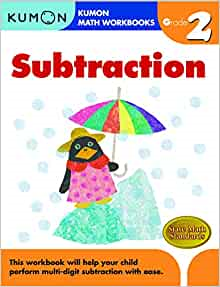• \$5.96
Unit price per
Shipping calculated at checkout.

This workbook helps your child subtract three-digit numbers with confidence. Children will learn to regroup (borrow) when subtracting, which equips them with the tools to subtract three-digit numbers. Vertical subtraction is included along with patterning and sequencing activities, so children gain a fundamental understanding of subtraction.

Topics Covered in this Book:

• Horizontal subtraction review
• Vertical form subtraction
• Regrouping (borrowing)
• Three-digit minus two-digit subtraction
• Early three- & four-digit subtraction
• Addition & subtraction of three two-digit numbers
• Patterns & sequencing

8 ½ x 11 inches. paperback. 80 pages. full color.

* Due to pandemic delays, please allow 2 weeks for items to ship